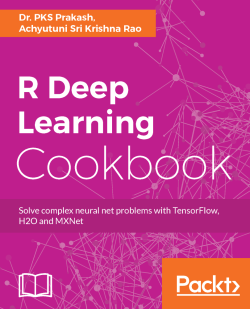# R Deep Learning Cookbook4 (2 reviews total)
By Dr. PKS Prakash , Achyutuni Sri Krishna Rao
• \$37.99 eBook
• \$46.99 Print + eBook
What do you get with a Packt Subscription?

• Constantly updated with 100+ new titles each month
• Breadth and depth in over 1,000+ technologies

Deep Learning is the next big thing. It is a part of machine learning. It's favorable results in applications with huge and complex data is remarkable. Simultaneously, R programming language is very popular amongst the data miners and statisticians.

This book will help you to get through the problems that you face during the execution of different tasks and Understand hacks in deep learning, neural networks, and advanced machine learning techniques. It will also take you through complex deep learning algorithms and various deep learning packages and libraries in R. It will be starting with different packages in Deep Learning to neural networks and structures. You will also encounter the applications in text mining and processing along with a comparison between CPU and GPU performance.

By the end of the book, you will have a logical understanding of Deep learning and different deep learning packages to have the most appropriate solutions for your problems.

Publication date:
August 2017
Publisher
Packt
Pages
288
ISBN
9781787121089

## Chapter 1. Getting Started

In this chapter, we will cover the following topics:

• Installing R with an IDE
• Installing a Jupyter Notebook application
• Starting with the basics of machine learning in R
• Setting up deep learning tools/packages in R
• Installing MXNet in R
• Installing TensorFlow in R
• Installing H2O in R
• Installing all three packages at once using Docker

## Introduction

This chapter will get you started with deep learning and help you set up your systems to develop deep learning models. The chapter is more focused on giving the audience a heads-up on what is expected from the book and the prerequisites required to go through the book. The current book is intended for students or professionals who want to quickly build a background in the applications of deep learning. The book will be more practical and application-focused using R as a tool to build deep learning models.

### Note

For a detailed theory on deep learning, refer to Deep Learning by Goodfellow et al. 2016. For a machine learning background refer Python Machine Learning by S. Raschka, 2015.

We will use the R programming language to demonstrate applications of deep learning. You are expected to have the following prerequisites throughout the book:

• Basic R programming knowledge
• Basic understanding of Linux; we will use the Ubuntu (16.04) operating system
• Basic understanding of machine learning concepts
• For Windows or macOS, a basic understanding of Docker

## Installing R with an IDE

Before we begin, let's install an IDE for R. For R the most popular IDEs are Rstudio and Jupyter. Rstudio is dedicated to R whereas Jupyter provide multi-language support including R. Jupyter also provides an interactive environment and allow you to combine code, text, and graphics into a single notebook.

R supports multiple operating systems such as Windows, macOS X, and Linux. The installation files for R can be downloaded from any one of the mirror sites at Comprehensive R Archive Network (CRAN) at https://cran.r-project.org/. The CRAN is also a major repository for packages in R. The programming language R is available under both 32-bit and 64-bit architectures.

### How to do it...

1. Of r-base-dev is also highly recommended as it has many inbuilt functions. It also enables the `install.packages()` command, which is used to compile and install new R packages directly from the CRAN using the R console. The default R console looks as follows: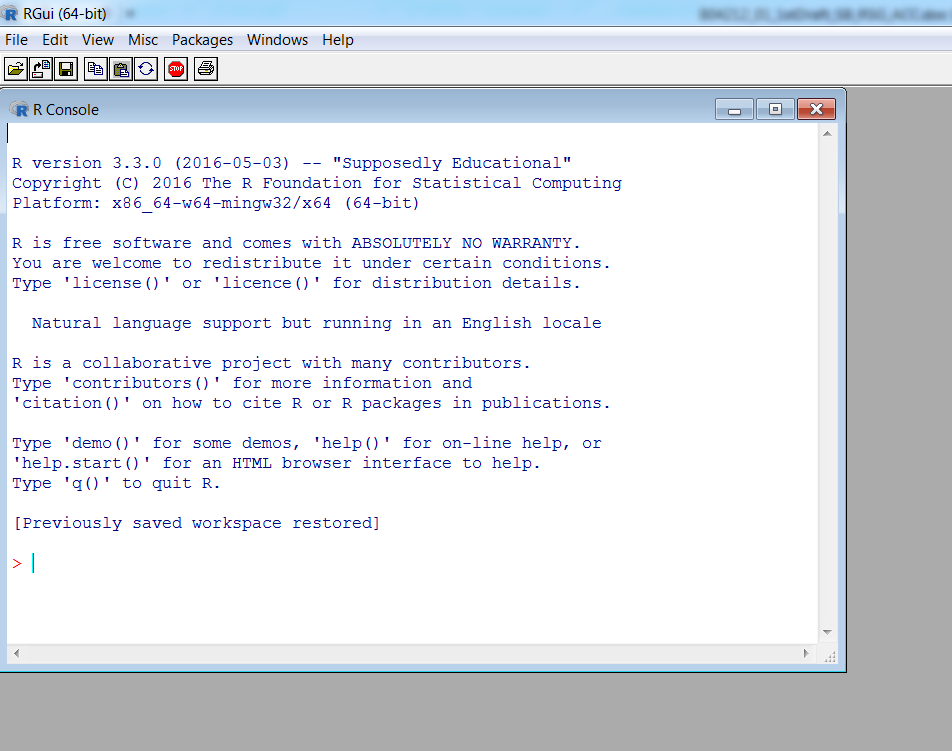Default R console

1. For programming purposes, an Integrated Development Environment (IDE) is recommended as it helps enhance productivity. One of the most popular open source IDEs for R is Rstudio. Rstudio also provides you with an Rstudio server, which facilitates a web-based environment to program in R. The interface for the Rstudio IDE is shown in the following screenshot: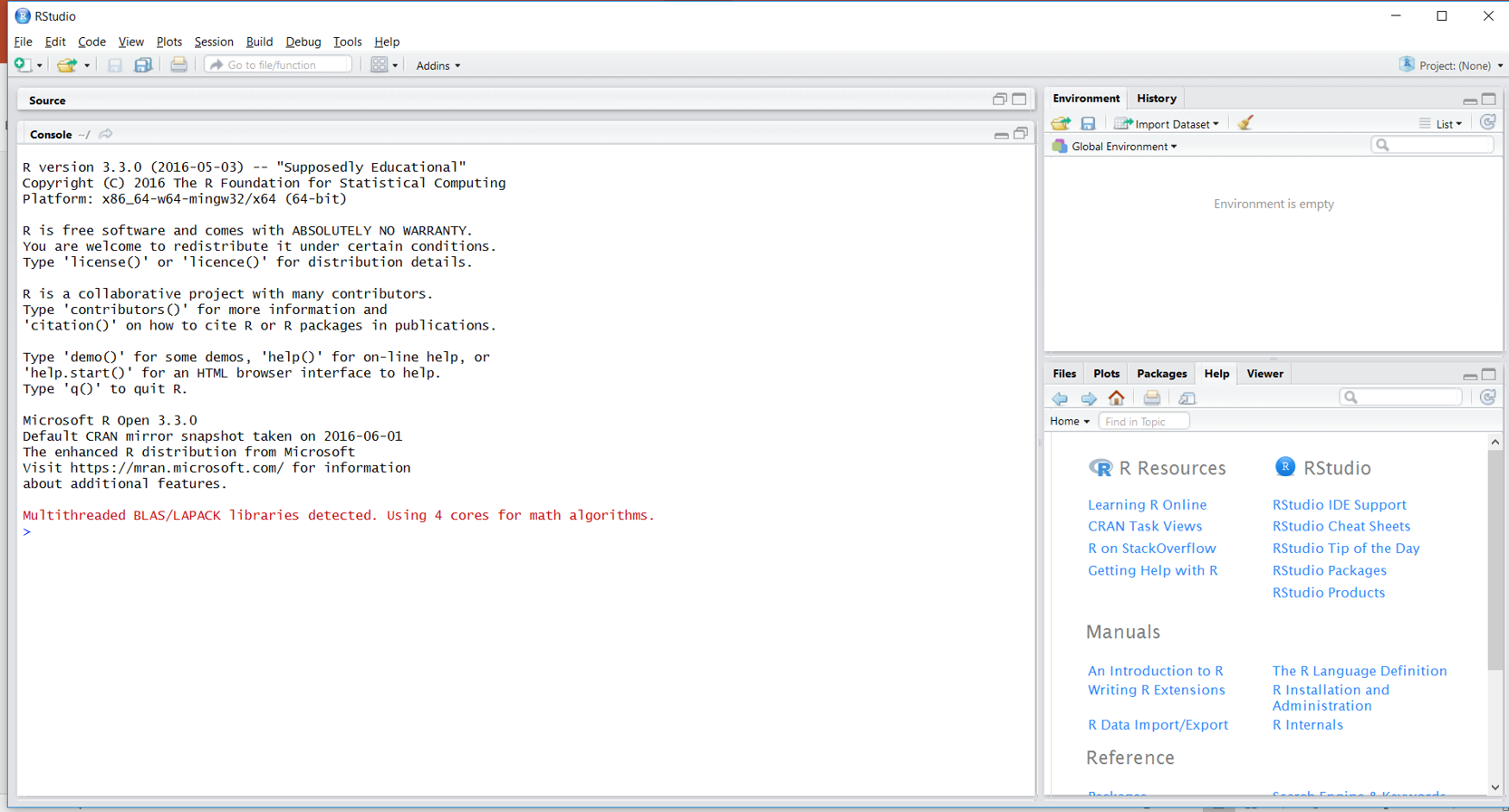Rstudio Integrated Development Environment for R

## Installing a Jupyter Notebook application

Another famous editor these days is the Jupyter Notebook app. This app produces notebook documents that integrate documentation, code, and analysis together. It supports many computational kernels including R. It is a server, client-side, web-based application that can be accessed using a browser.

### How to do it...

Jupyter Notebook can be installed using the following steps:

1. Jupyter Notebook can be installed using `pip`:
```pip3 install --upgrade pip
pip3 install jupyter```
1. If you have installed Anaconda, then the default computational kernel installed is Python. To install an R computation kernel in Jupyter within the same environment, type the following command in a terminal:
`conda install -c r r-essentials`
1. To install the R computational kernel in a new environment named `new-env` within conda, type as follows:
`conda create -n new-env -c r r-essentials`
1. Another way to include the R computational kernel in Jupyter Notebook uses the `IRkernel` package. To install through this process, start the R IDE. The first step is to install dependencies required for the `IRkernal` installation:
```chooseCRANmirror(ind=55) # choose mirror for installation
install.packages(c('repr', 'IRdisplay', 'crayon', 'pbdZMQ',
'devtools'), dependencies=TRUE)```
1. Once all the dependencies are installed from CRAN, install the `IRkernal` package from GitHub:
```library(devtools)
library(methods)
options(repos=c(CRAN='https://cran.rstudio.com'))
devtools::install_github('IRkernel/IRkernel')```
1. Once all the requirements are satisfied, the R computation kernel can be set up in Jupyter Notebook using the following script:
```library(IRkernel)
IRkernel::installspec(name = 'ir32', displayname = 'R 3.2')```
1. Jupyter Notebook can be started by opening a shell/terminal. Run the following command to start the Jupyter Notebook interface in the browser, as shown in the screenshot following this code:
`jupyter notebook`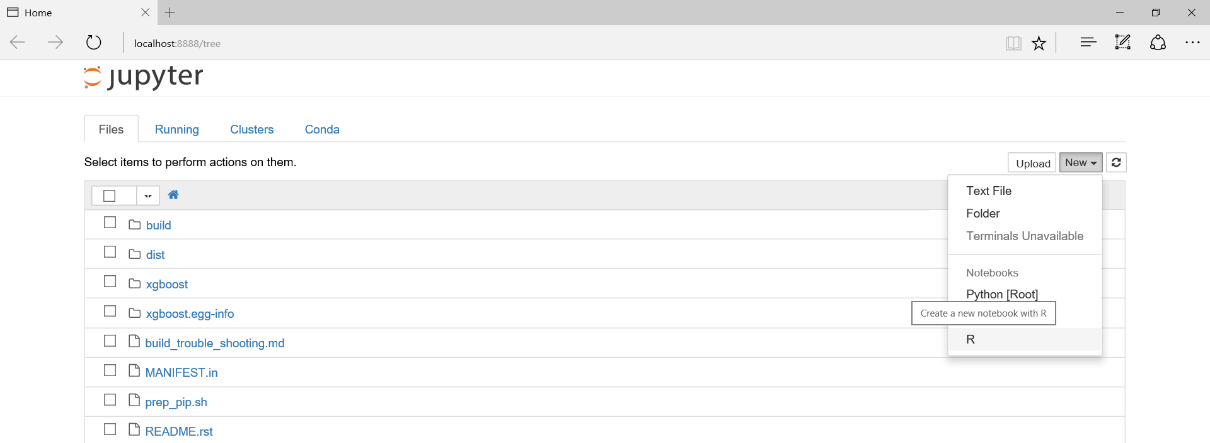Jupyter Notebook with the R computation engine

### There's more...

R, as with most of the packages utilized in this book, is supported by most operating systems. However, you can make use of Docker or VirtualBox to set up a working environment similar to the one used in this book.

For Docker installation and setup information, refer to https://docs.docker.com/ and select the Docker image appropriate to your operating system. Similarly, VirtualBox binaries can be downloaded and installed at https://www.virtualbox.org/wiki/Downloads.

## Starting with the basics of machine learning in R

Deep learning is a subcategory of machine learning inspired by the structure and functioning of a human brain. In recent times, deep learning has gained a lot of traction primarily because of higher computational power, bigger datasets, and better algorithms with (artificial) intelligent learning abilities and more inquisitiveness for data-driven insights. Before we get into the details of deep learning, let's understand some basic concepts of machine learning that form the basis for most analytical solutions.

Machine learning is an arena of developing algorithms with the ability to mine natural patterns from the data such that better decisions are made using predictive insights. These insights are pertinent across a horizon of real-world applications, from medical diagnosis (using computational biology) to real-time stock trading (using computational finance), from weather forecasting to natural language processing, from predictive maintenance (in automation and manufacturing) to prescriptive recommendations (in e-commerce and e-retail), and so on.

The following figure elucidates two primary techniques of machine learning; namely, supervised learning and unsupervised learning: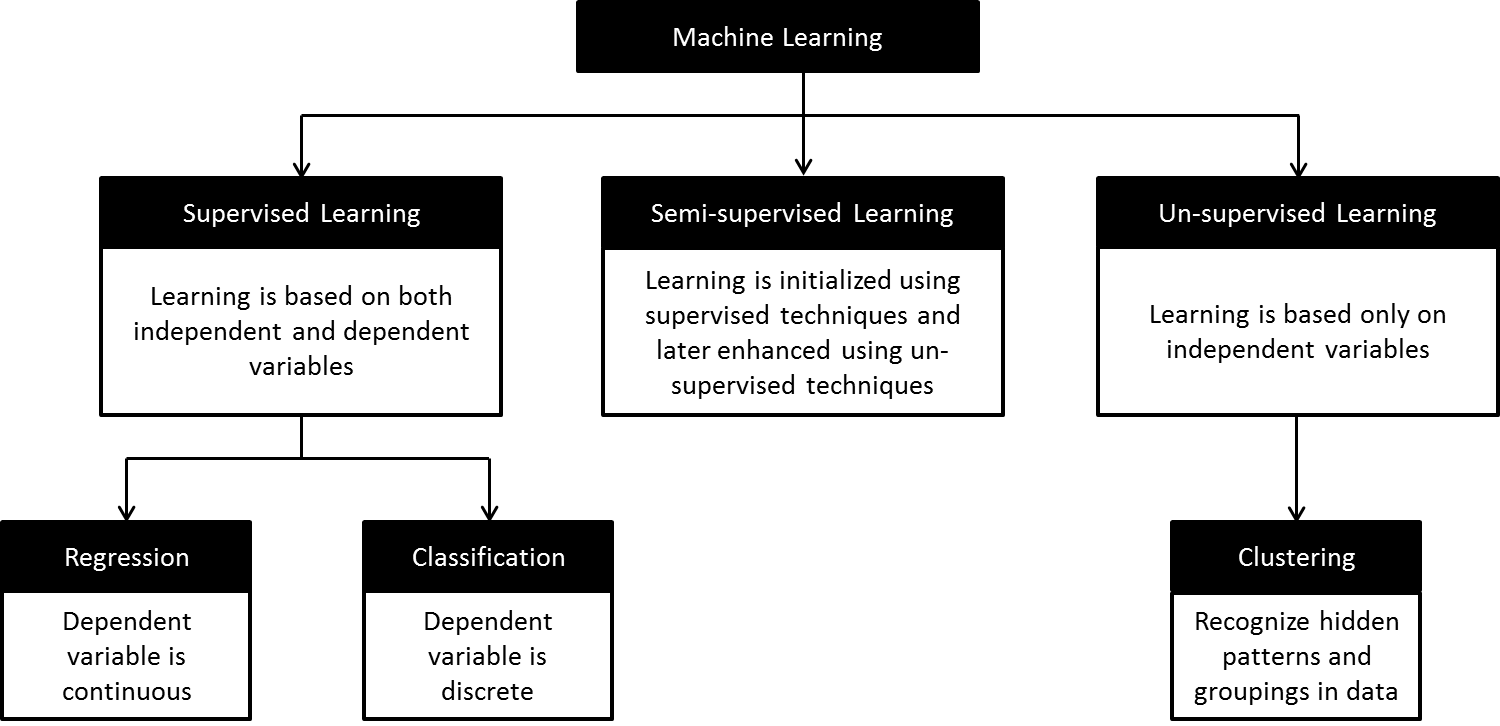Classification of different techniques in machine learning

Supervised learning: Supervised learning is a form of evidence-based learning. The evidence is the known outcome for a given input and is in turn used to train the predictive model. Models are further classified into regression and classification, based on the outcome data type. In the former, the outcome is continuous, and in the latter the outcome is discrete. Stock trading and weather forecasting are some widely used applications of regression models, and span detection, speech recognition, and image classification are some widely used applications of classification models.

Some algorithms for regression are linear regression, Generalized Linear Models (GLM), Support Vector Regression (SVR), neural networks, decision trees, and so on; in classification, we have logistic regression, Support Vector Machines (SVM), Linear discriminant analysis (LDA), Naive Bayes, nearest neighbors, and so on.

Semi-supervised learning: Semi-supervised learning is a class of supervised learning using unsupervised techniques. This technique is very useful in scenarios where the cost of labeling an entire dataset is highly impractical against the cost of acquiring and analyzing unlabeled data.

Unsupervised learning: As the name suggests, learning from data with no outcome (or supervision) is called unsupervised learning. It is a form of inferential learning based on hidden patterns and intrinsic groups in the given data. Its applications include market pattern recognition, genetic clustering, and so on.

Some widely used clustering algorithms are k-means, hierarchical, k-medoids, Fuzzy C-means, hidden markov, neural networks, and many more.

### How to do it...

Let's take a look at linear regression in supervised learning:

1. Let's begin with a simple example of linear regression where we need to determine the relationship between men's height (in cms) and weight (in kgs). The following sample data represents the height and weight of 10 random men:
```data <- data.frame("height" = c(131, 154, 120, 166, 108, 115,
158, 144, 131, 112),
"weight" = c(54, 70, 47, 79, 36, 48, 65,
63, 54, 40))```
1. Now, generate a linear regression model as follows:
`lm_model <- lm(weight ~ height, data)`
1. The following plot shows the relationship between men's height and weight along with the fitted line:
```plot(data, col = "red", main = "Relationship between height and
weight",cex = 1.7, pch = 1, xlab = "Height in cms", ylab = "Weight
in kgs")
abline(lm(weight ~ height, data))```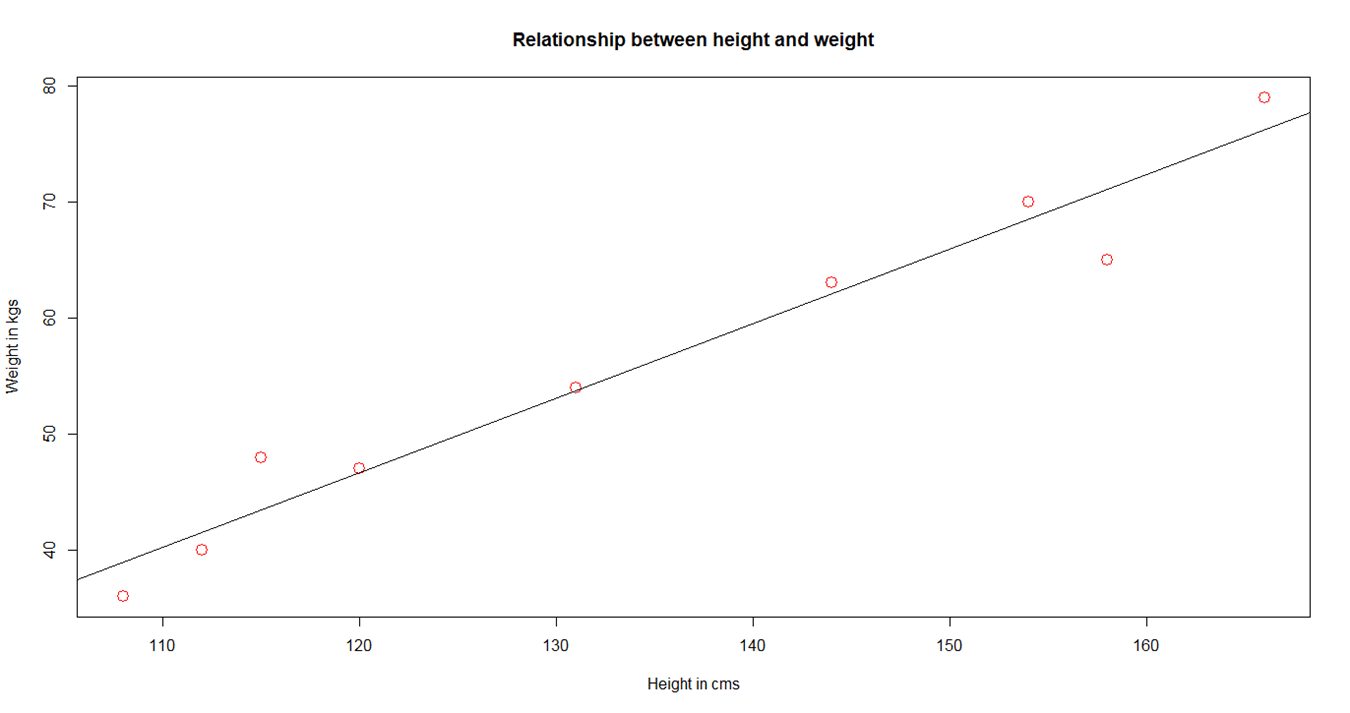Linear relationship between weight and height

1. In semi-supervised models, the learning is primarily initiated using labeled data (a smaller quantity in general) and then augmented using unlabeled data (a larger quantity in general).

Let's perform K-means clustering (unsupervised learning) on a widely used dataset, iris.

1. This dataset consists of three different species of iris (Setosa, Versicolor, and Virginica) along with their distinct features such as sepal length, sepal width, petal length, and petal width:
```data(iris)
Sepal.Length Sepal.Width Petal.Length Petal.Width Species
1 5.1 3.5 1.4 0.2 setosa
2 4.9 3.0 1.4 0.2 setosa
3 4.7 3.2 1.3 0.2 setosa
4 4.6 3.1 1.5 0.2 setosa
5 5.0 3.6 1.4 0.2 setosa
6 5.4 3.9 1.7 0.4 setosa```
1. The following plots show the variation of features across irises. Petal features show a distinct variation as against sepal features:
```library(ggplot2)
library(gridExtra)
plot1 <- ggplot(iris, aes(Sepal.Length, Sepal.Width, color =
Species))
geom_point(size = 2)
ggtitle("Variation by Sepal features")
plot2 <- ggplot(iris, aes(Petal.Length, Petal.Width, color =
Species))
geom_point(size = 2)
ggtitle("Variation by Petal features")
grid.arrange(plot1, plot2, ncol=2)```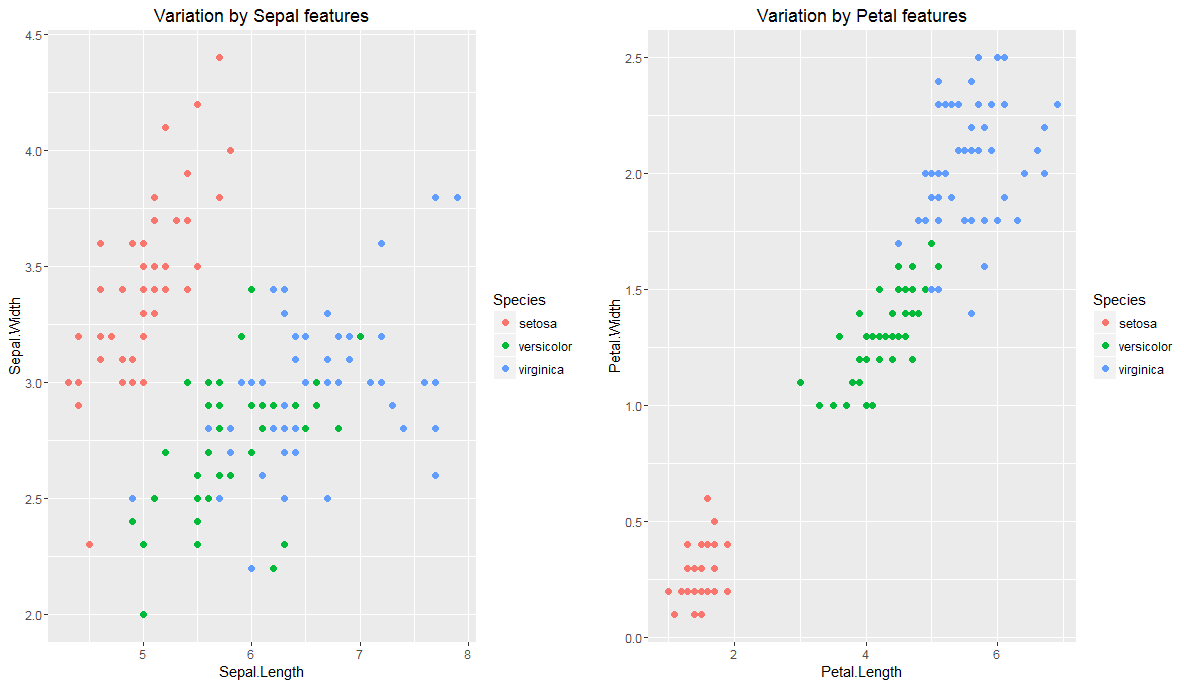Variation of sepal and petal features by length and width

1. As petal features show a good variation across irises, let's perform K-means clustering using the petal length and petal width:
```set.seed(1234567)
iris.cluster <- kmeans(iris[, c("Petal.Length","Petal.Width")],
3, nstart = 10)
iris.cluster\$cluster <- as.factor(iris.cluster\$cluster)```
1. The following code snippet shows a cross-tab between clusters and species (irises). We can see that cluster 1 is primarily attributed with setosa, cluster 2 with versicolor, and cluster 3 with virginica:
```> table(cluster=iris.cluster\$cluster,species= iris\$Species)
species
cluster setosa versicolor virginica
1 50 0 0
2 0 48 4
3 0 2 46

ggplot(iris, aes(Petal.Length, Petal.Width, color =
iris.cluster\$cluster)) + geom_point() + ggtitle("Variation by
Clusters")```
1. The following plot shows the distribution of clusters: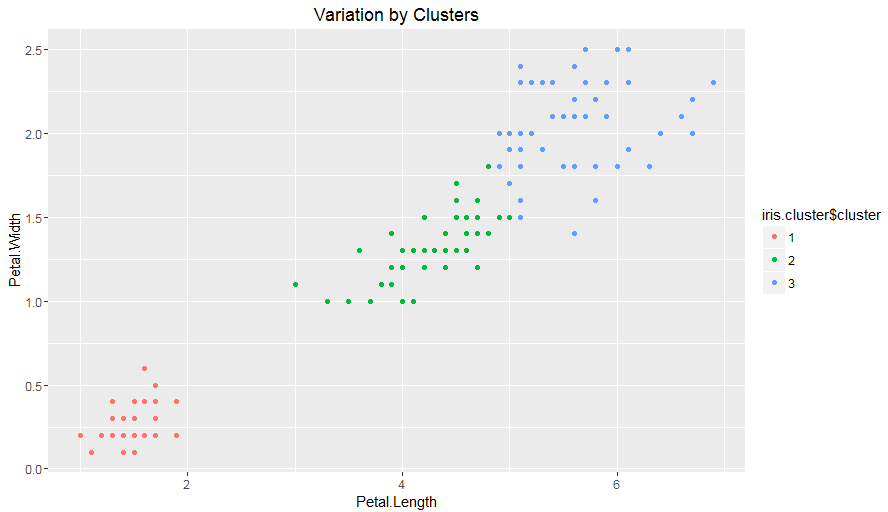Variation of irises across three clusters

### How it works...

Model evaluation is a key step in any machine learning process. It is different for supervised and unsupervised models. In supervised models, predictions play a major role; whereas in unsupervised models, homogeneity within clusters and heterogeneity across clusters play a major role.

Some widely used model evaluation parameters for regression models (including cross validation) are as follows:

• Coefficient of determination
• Root mean squared error
• Mean absolute error
• Akaike or Bayesian information criterion

Some widely used model evaluation parameters for classification models (including cross validation) are as follows:

• Confusion matrix (accuracy, precision, recall, and F1-score)
• Gain or lift charts
• Area under ROC (receiver operating characteristic) curve
• Concordant and discordant ratio

Some of the widely used evaluation parameters of unsupervised models (clustering) are as follows:

• Contingency tables
• Sum of squared errors between clustering objects and cluster centers or centroids
• Silhouette value
• Rand index
• Matching index
• Pairwise and adjusted pairwise precision and recall (primarily used in NLP)

Bias and variance are two key error components of any supervised model; their trade-off plays a vital role in model tuning and selection. Bias is due to incorrect assumptions made by a predictive model while learning outcomes, whereas variance is due to model rigidity toward the training dataset. In other words, higher bias leads to underfitting and higher variance leads to overfitting of models.

In bias, the assumptions are on target functional forms. Hence, this is dominant in parametric models such as linear regression, logistic regression, and linear discriminant analysis as their outcomes are a functional form of input variables.

Variance, on the other hand, shows how susceptible models are to change in datasets. Generally, target functional forms control variance. Hence, this is dominant in non-parametric models such as decision trees, support vector machines, and K-nearest neighbors as their outcomes are not directly a functional form of input variables. In other words, the hyperparameters of non-parametric models can lead to overfitting of predictive models.

## Setting up deep learning tools/packages in R

The major deep learning packages are developed in C/C++ for efficiency purposes and wrappers are developed in R to efficiently develop, extend, and execute deep learning models.

A lot of open source deep learning libraries are available. The prominent libraries in this area are as follows:

• Theano
• TensorFlow
• Torch
• Caffe

There are other prominent packages available on the market such as H2O, CNTK (Microsoft Cognitive Toolkit), darch, Mocha, and ConvNetJS. There are a lot of wrappers that are developed around these packages to support the easy development of deep learning models, such as Keras and Lasagne in Python and MXNet, both supporting multiple languages.

### How to do it...

1. This chapter will cover the MXNet and TensorFlow packages (developed in C++ and CUDA for a highly optimized performance in GPU).
2. Additionally, the `h2o` package will be used to develop some deep learning models. The `h2o` package in R is implemented as a REST API, which connects to the H2O server (it runs as Java Virtual Machines (JVM)). We will provide quick setup instructions for these packages in the following sections

## Installing MXNet in R

This section will cover the installation of MXNet in R.

The MXNet package is a lightweight deep learning architecture supporting multiple programming languages such as R, Python, and Julia. From a programming perspective, it is a combination of symbolic and imperative programming with support for CPU and GPU.

The CPU-based MXNet in R can be installed using the prebuilt binary package or the source code where the libraries need to be built. In Windows/mac, prebuilt binary packages can be download and installed directly from the R console. MXNet requires the R version to be 3.2.0 and higher. The installation requires the `drat` package from CRAN. The `drat` package helps maintain R repositories and can be installed using the `install.packages()` command.

To install MXNet on Linux (13.10 or later), the following are some dependencies:

• Git (to get the code from GitHub)
• libatlas-base-dev (to perform linear algebraic operations)
• libopencv-dev (to perform computer vision operations)

To install MXNet with a GPU processor, the following are some dependencies:

• Microsoft Visual Studio 2013
• The NVIDIA CUDA Toolkit
• The MXNet package
• cuDNN (to provide a deep neural network library)

Another quick way to install `mxnet` with all the dependencies is to use the prebuilt Docker image from the `chstone` repository. The `chstone`/`mxnet-gpu` Docker image will be installed using the following tools:

• MXNet for R and Python
• Ubuntu 16.04
• CUDA (Optional for GPU)
• cuDNN (Optional for GPU)

### How to do it...

1. The following R command installs MXNet using prebuilt binary packages, and is hassle-free. The `drat` package is then used to add the `dlmc` repository from git followed by the `mxnet` installation:
```install.packages("drat", repos="https://cran.rstudio.com")
install.packages("mxnet")```

2. The following code helps install MXNet in Ubuntu (V16.04). The first two lines are used to install dependencies and the remaining lines are used to install MXNet, subject to the satisfaction of all the dependencies:

```sudo apt-get update
sudo apt-get install -y build-essential git libatlas-base-dev
libopencv-dev
git clone https://github.com/dmlc/mxnet.git ~/mxnet --recursive
cd ~/mxnet
cp make/config.mk .
echo "USE_BLAS=openblas" >>config.mk
make -j\$(nproc)```

3. If MXNet is to be built for GPU, the following `config` needs to be updated before the `make` command:

```echo "USE_CUDA=1" >>config.mk
echo "USE_CUDA_PATH=/usr/local/cuda" >>config.mk
echo "USE_CUDNN=1" >>config.mk```

### Note

A detailed installation of MXNet for other operating systems can be found at http://mxnet.io/get_started/setup.html.

4. The following command is used to install MXNet (GPU-based) using Docker with all the dependencies:

`docker pull chstone/mxnet-gpu`

## Installing TensorFlow in R

This section will cover another very popular open source machine learning package, TensorFlow, which is very effective in building deep learning models.

TensorFlow is another open source library developed by the Google Brain Team to build numerical computation models using data flow graphs. The core of TensorFlow was developed in C++ with the wrapper in Python. The `tensorflow` package in R gives you access to the TensorFlow API composed of Python modules to execute computation models. TensorFlow supports both CPU- and GPU-based computations.

The `tensorflow` package in R calls the Python tensorflow API for execution, which is essential to install the `tensorflow` package in both R and Python to make R work. The following are the dependencies for `tensorflow`:

• Python 2.7 / 3.x
• R (>3.2)
• devtools package in R for installing TensorFlow from GitHub
• TensorFlow in Python
• pip

### How to do it...

1. Once all the mentioned dependencies are installed, `tensorflow` can be installed from `devtools` directly using the `install_github` command as follows:
`devtools::install_github("rstudio/tensorflow")`
1. Before loading `tensorflow` in R, you need to set up the path for Python as the system environment variable. This can be done directly from the R environment, as shown in the following command:
```Sys.setenv(TENSORFLOW_PYTHON="/usr/bin/python")
library(tensorflow)```

If the Python `tensorflow` module is not installed, R will give the following error: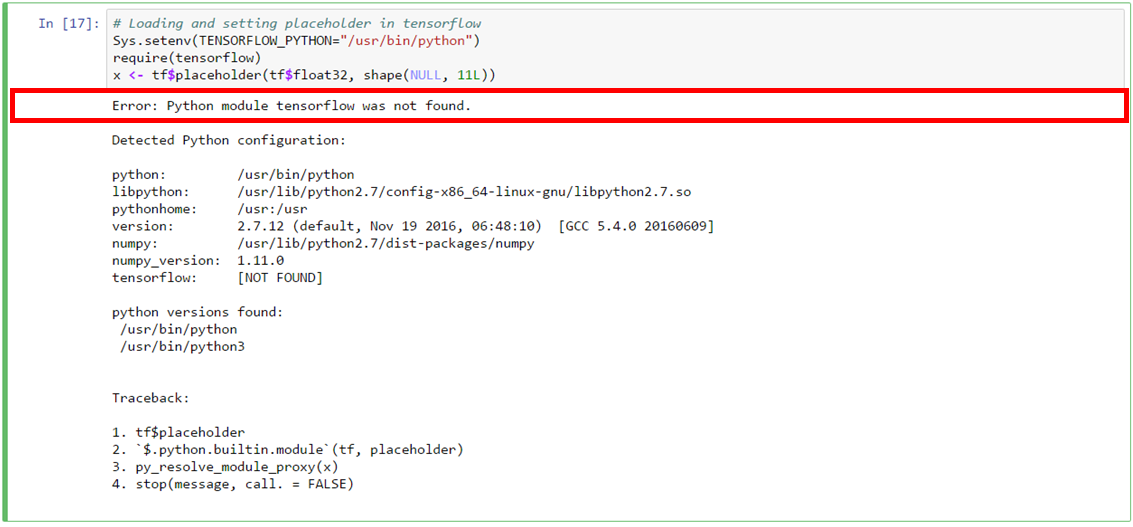Error raised by R if tensorflow in Python is not installed

`tensorflow` in Python can be installed using `pip`:

```pip install tensorflow # Python 2.7 with no GPU support
pip3 install tensorflow # Python 3.x with no GPU support
pip install tensorflow-gpu # Python 2.7 with GPU support
pip3 install tensorflow-gpu # Python 3.x with GPU support```

### How it works...

TensorFlow follows directed graph philosophy to set up computational models where mathematical operations are represented as nodes with each node supporting multiple input and output while the edges represent the communication of data between nodes. There are also edges known as control dependencies in TensorFlow that do not represent the data flow; rather the provide information related to control dependence such as node for the control dependence must finish processing before the destination node of control dependence starts executing.

An example TensorFlow graph for logistic regression scoring is shown in the following diagram: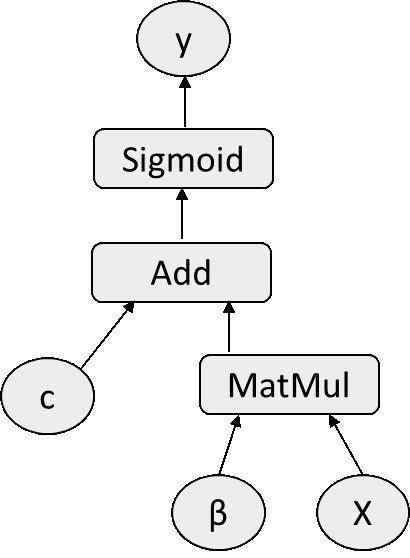TensorFlow graph for logistic regression

The preceding figure illustrates the TensorFlow graph to score logistic regression with optimized weights:The MatMul node performs matrix multiplication between input feature matrix X and optimized weight β. The constant C is then added to the output from the MatMul node. The output from Add is then transformed using the Sigmoid function to output Pr(y=1|X).

Get started with TensorFlow in R using resources at https://rstudio.github.io/tensorflow/.

## Installing H2O in R

H2O is another very popular open source library to build machine learning models. It is produced by H2O.ai and supports multiple languages including R and Python. The H2O package is a multipurpose machine learning library developed for a distributed environment to run algorithms on big data.

To set up H2O, the following systems are required:

• 64-bit Java Runtime Environment (version 1.6 or later)
• Minimum 2 GB RAM

H2O from R can be called using the `h2o` package. The `h2o` package has the following dependencies:

• RCurl
• rjson
• statmod
• survival
• stats
• tools
• utils
• methods

For machines that do not have curl-config installed, the RCurl dependency installation will fail in R and curl-config needs to be installed outside R.

### How to do it...

1. H2O can be installed directly from CRAN with the dependency parameter TRUE to install all CRAN-related `h2o` dependencies. This command will install all the R dependencies required for the `h2o` package:
`install.packages("h2o", dependencies = T)`
1. The following command is used to call the `h2o` package in the current R environment. The first-time execution of the `h2o` package will automatically download the JAR file before launching H2O, as shown in the following figure:
```library(h2o)
localH2O = h2o.init()```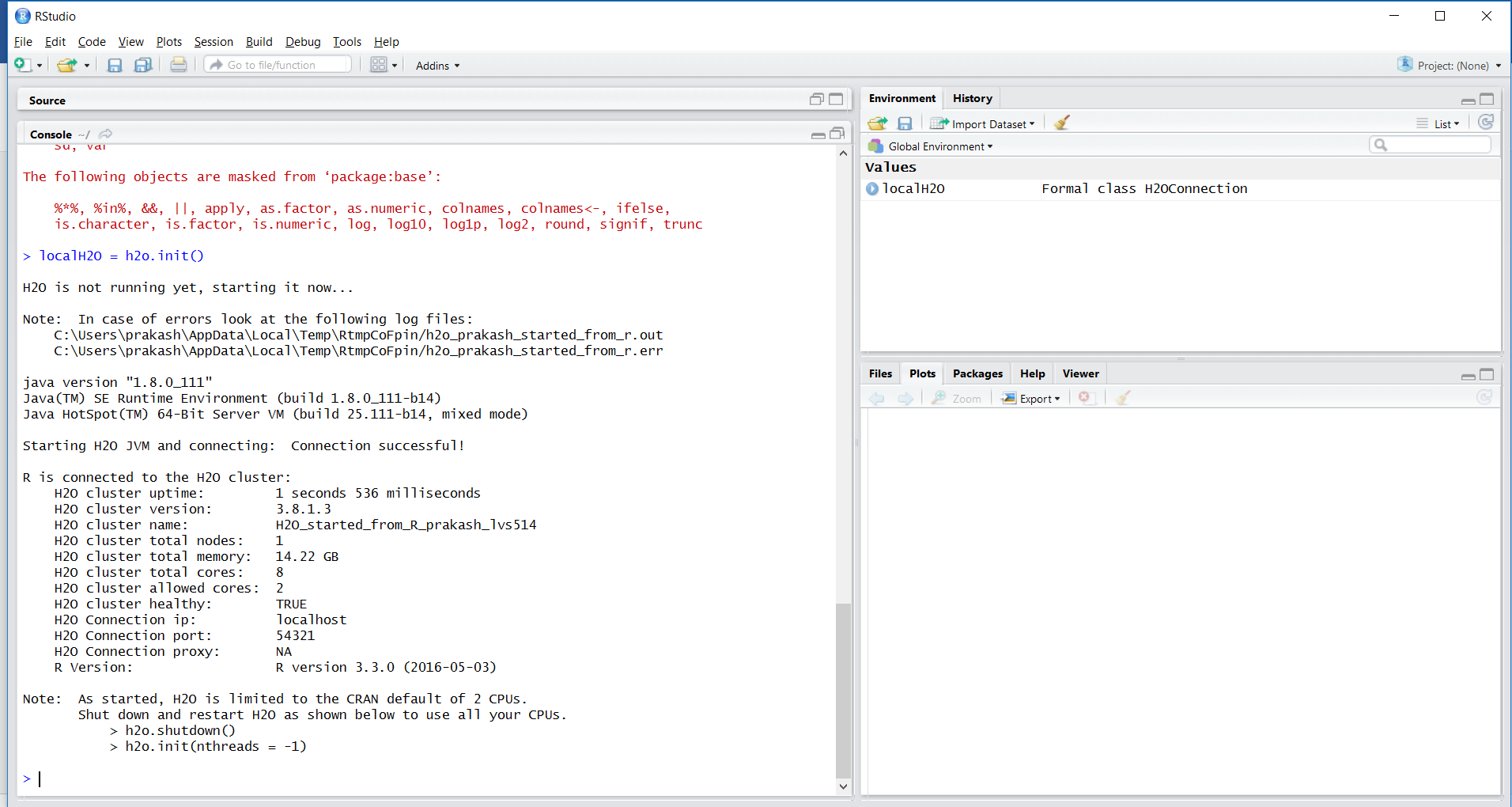Starting H2O cluster

1. The H2O cluster can be accessed using clusterip and port information. The current H2O cluster is running on localhost at port `54321`, as shown in the following screenshot: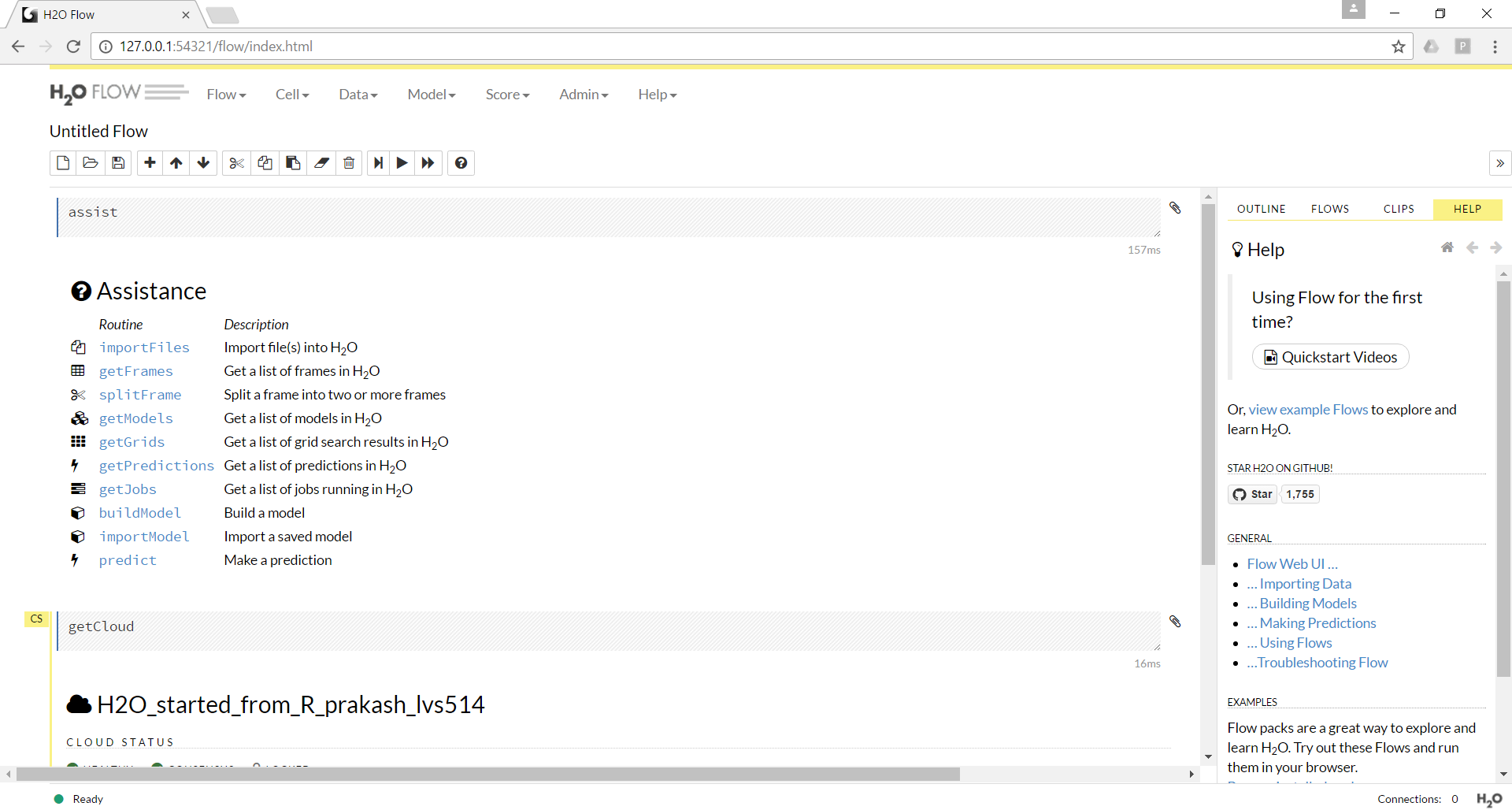H2O cluster running in the browser

### Note

Models in H2O can be developed interactively using a browser or scripting from R. H2O modeling is like creating a Jupyter Notebook but you create a flow with different operations such as importing data, splitting data, setting up a model, and scoring.

### How it works...

Let's build a logistic regression interactively using the H2O browser.

1. Start a new flow, as shown in the following screenshot: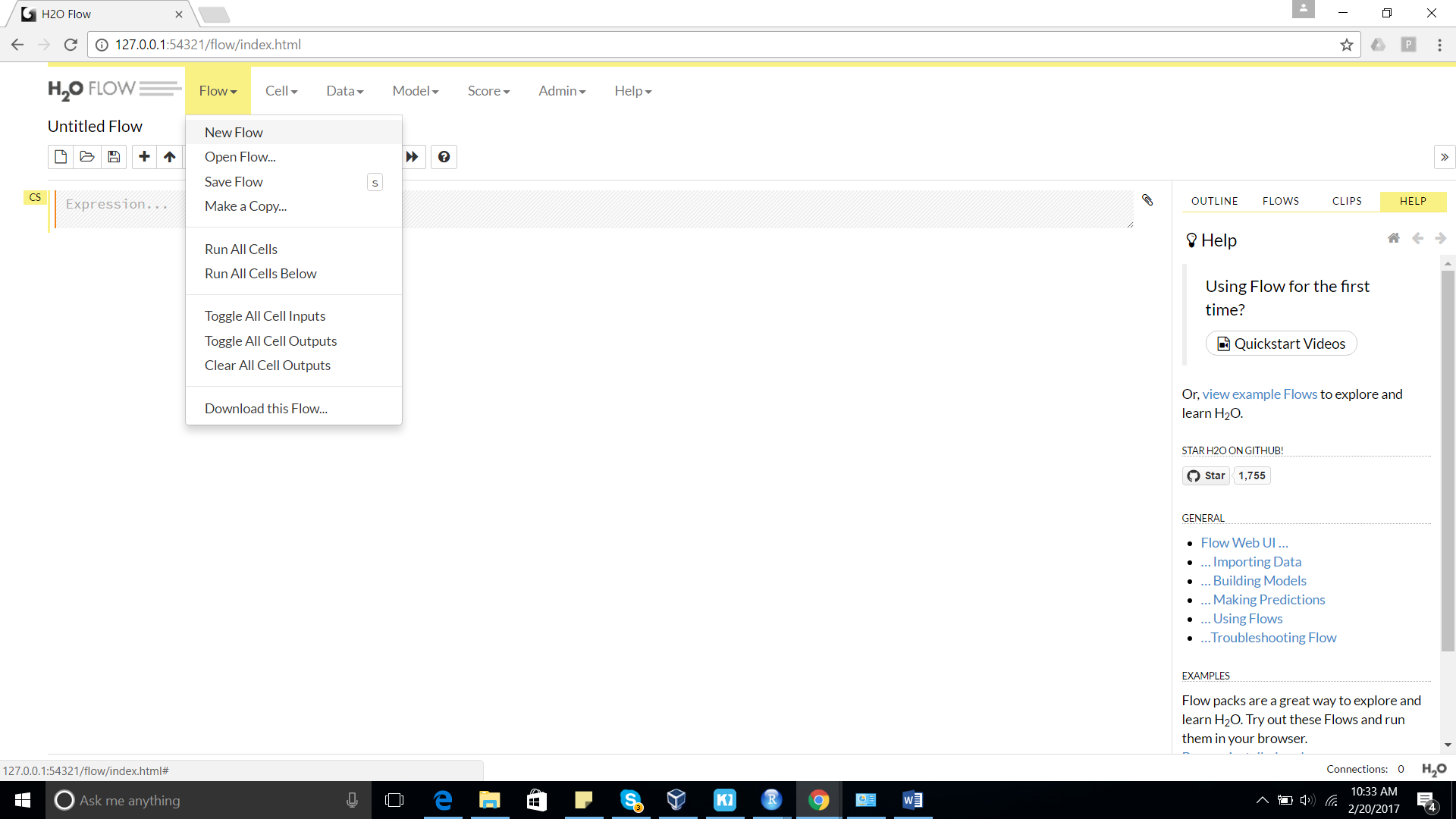Creating a new flow in H2O

1. Import a dataset using the Data menu, as shown in the following screenshot: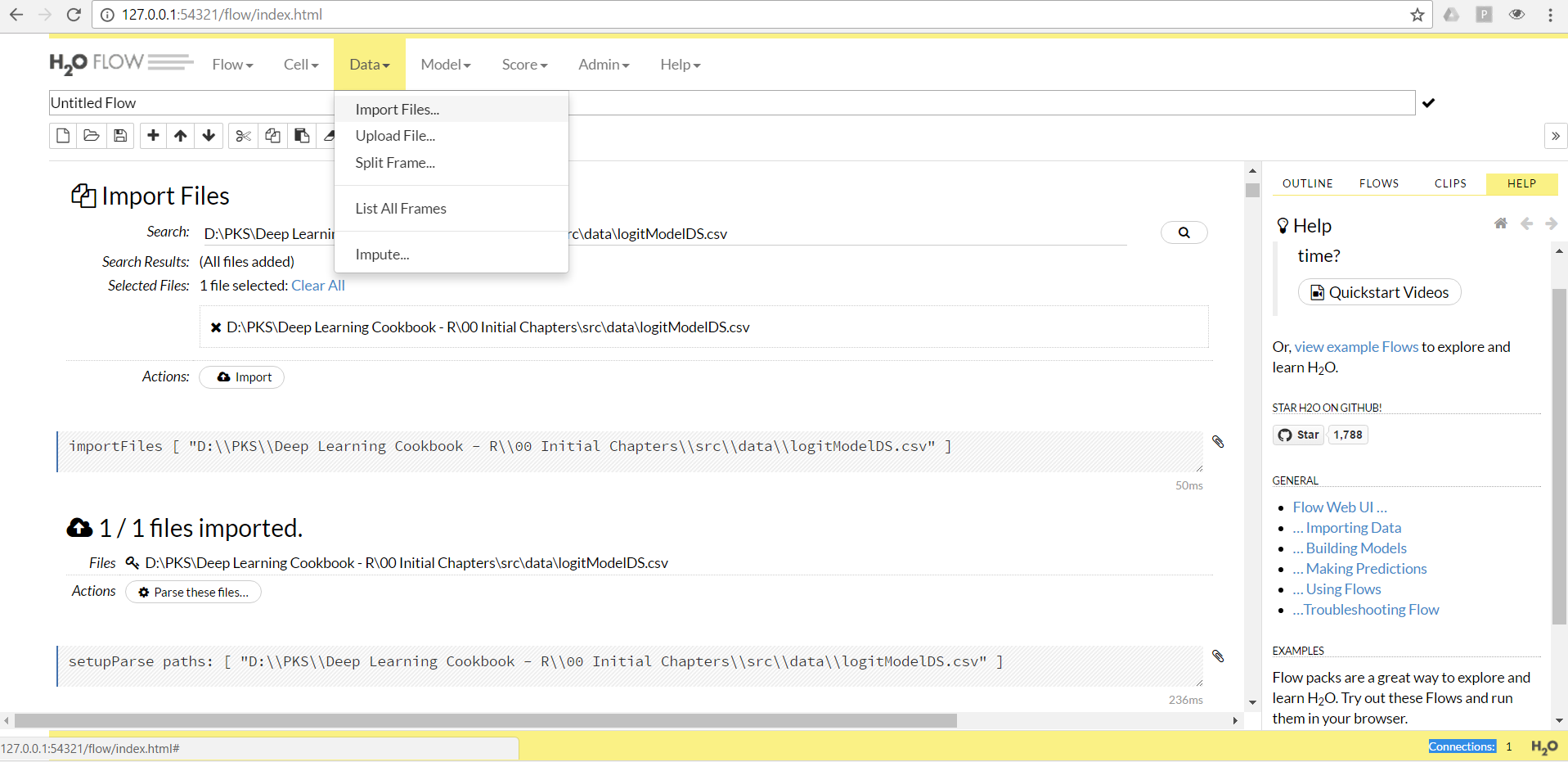Importing files to the H2O environment

1. The imported file in H2O can be parsed into the hex format (the native file format for H2O) using the Parse these files action, which will appear once the file is imported to the H2O environment: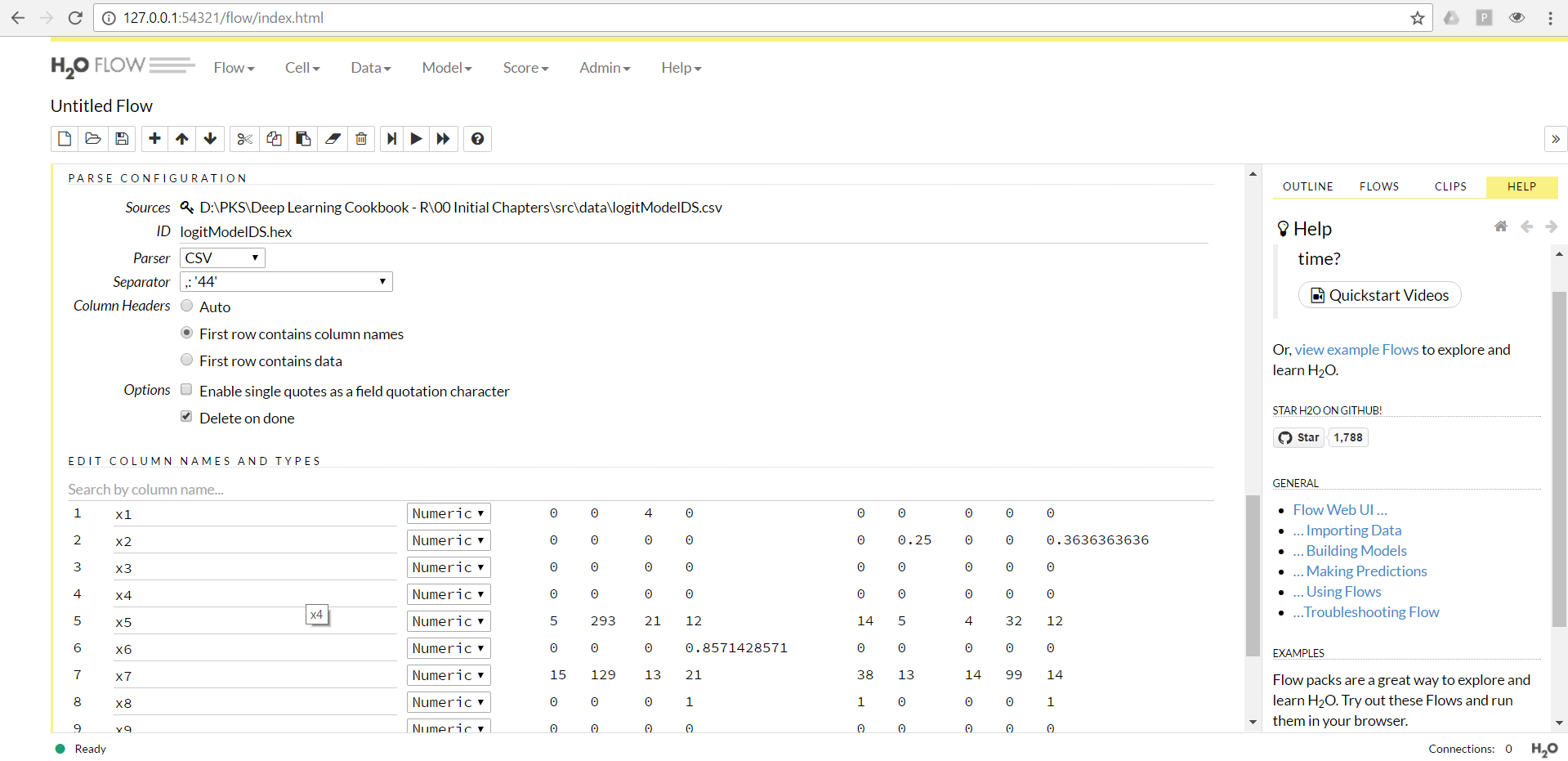Parsing the file to the hex format

1. The parsed data frame in H2O can be split into training and validation using the `Data` |`Split Frame` action, as shown in the following screenshot: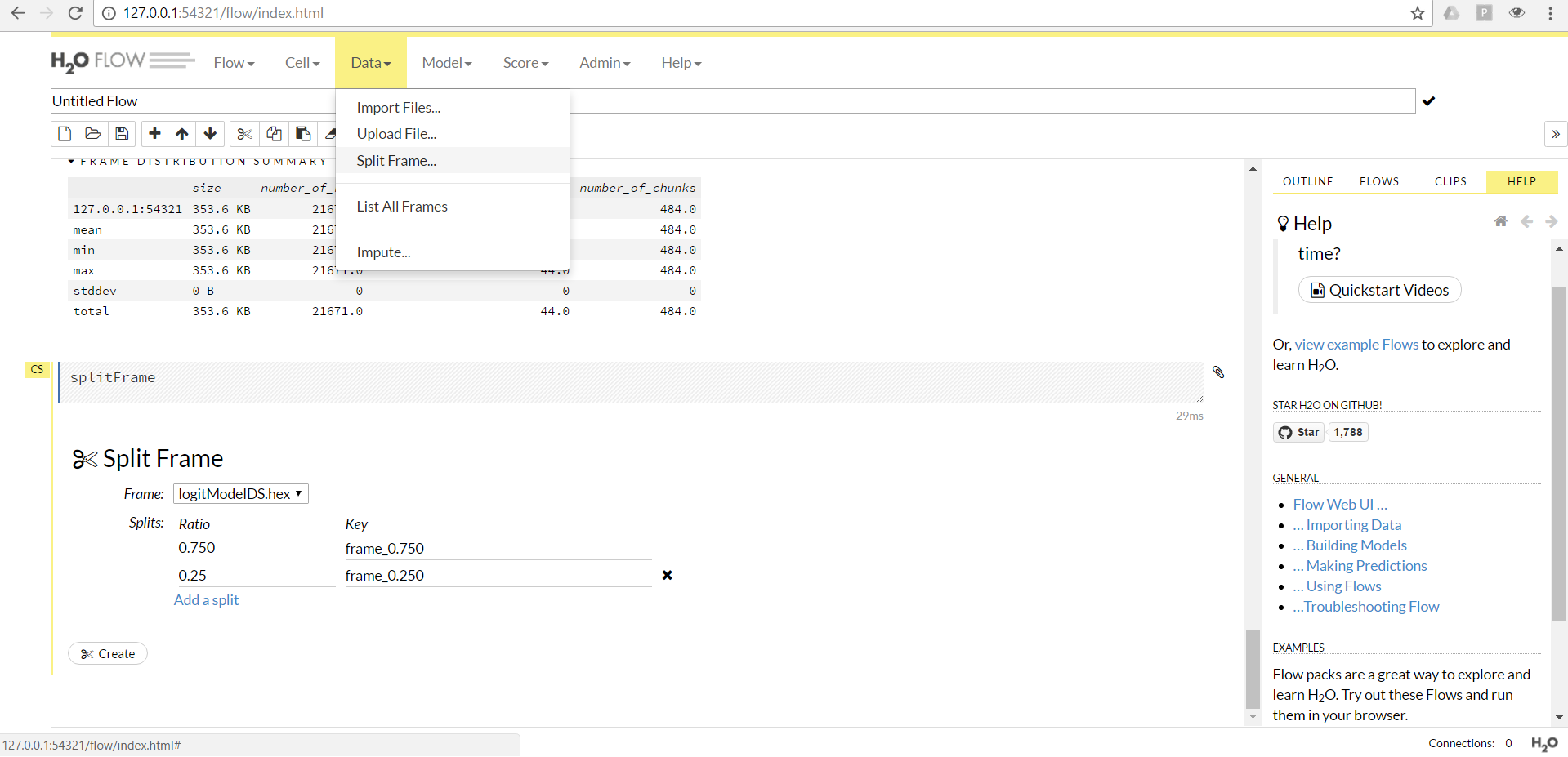Splitting the dataset into training and validation

1. Select the model from the `Model menu and set up the model-related parameters. An example for a glm model is seen in the following screenshot:`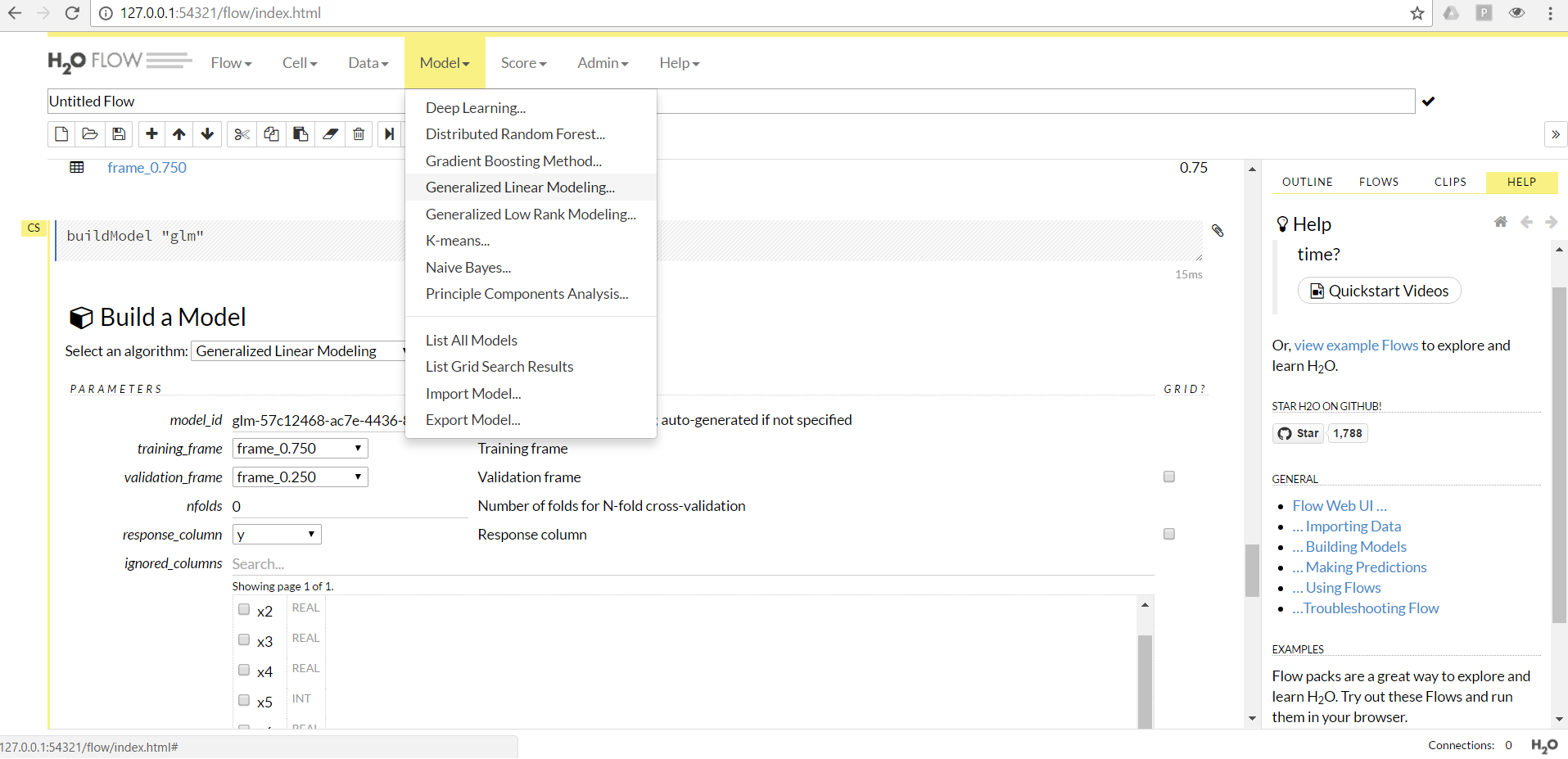Building a model in H2O

1. The `Score`|`predict` action can be used to score another hex data frame in H2O: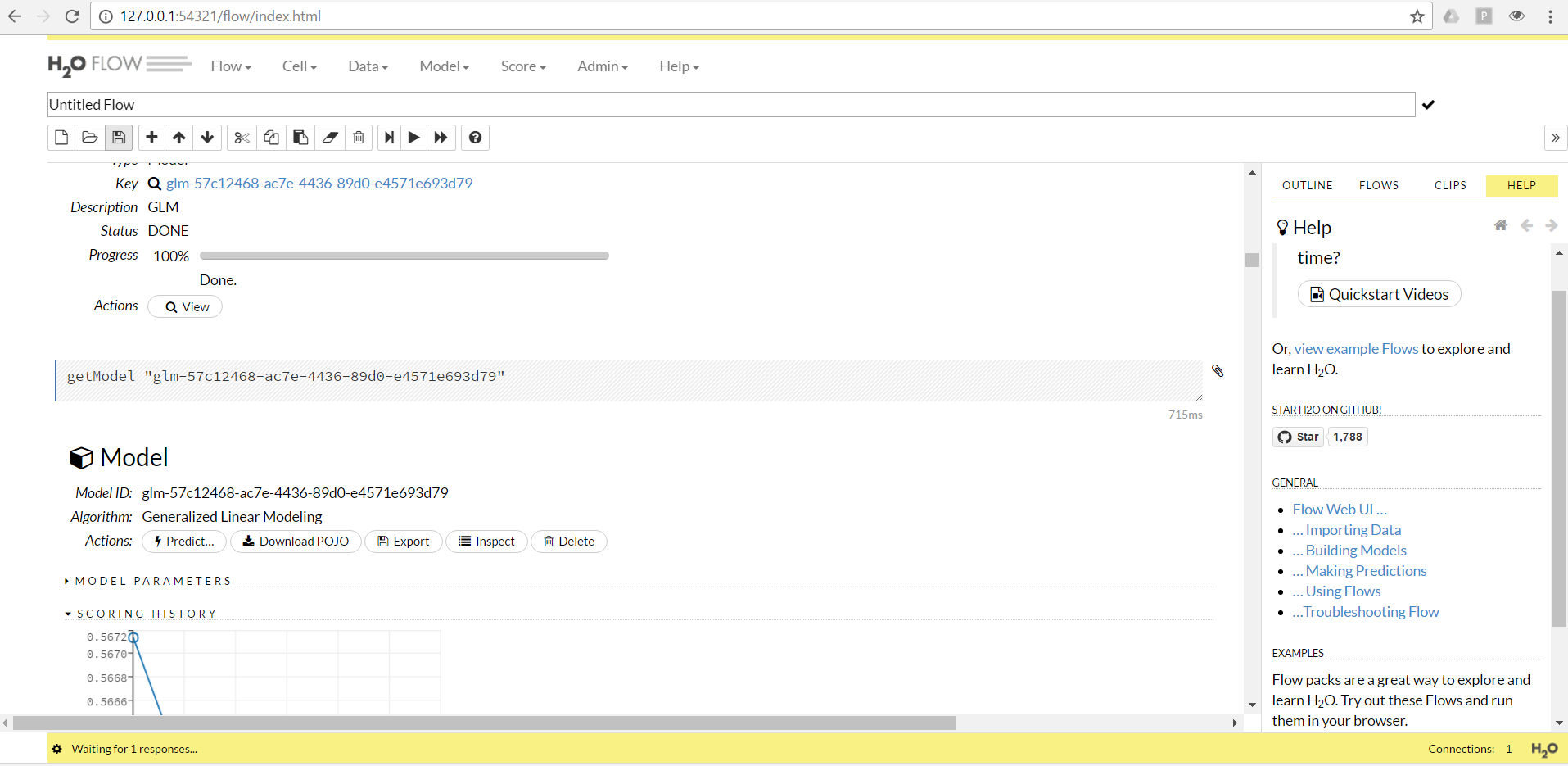Scoring in H2O

### There's more...

For more complicated scenarios that involve a lot of preprocessing, H2O can be called from R directly. This book will focus more on building models using H2O from R directly. If H2O is set up at a different location instead of localhost, then it can be connected within R by defining the correct `ip` and `port` at which the cluster is running:

`localH2O = h2o.init(ip = "localhost", port = 54321, nthreads = -1) `

Another critical parameter is the number of threads to be used to build the model; by default, n threads are set to -2, which means that two cores will be used. The value of -1 for n threads will make use of all available cores.

### Note

http://docs.h2o.ai/h2o/latest-stable/index.html#gettingstarted is very good using H2O in interactive mode.

## Installing all three packages at once using Docker

Docker is a software-contained platform that is used to host multiple software or apps side by side in isolated containers to get better computing density. Unlike virtual machines, containers are built only using libraries and the settings required by any software but do not bundle the entire operating system, thus making it lightweight and efficient.

Setting up all three packages could be quite cumbersome depending on the operating system utilized. The following dockerfile code can be used to set up an environment with `tensorflow`, `mxnet` with GPU, and `h2o` installed with all the dependencies:

```FROM chstone/mxnet-gpu:latest
MAINTAINER PKS Prakash <prakash5801>

# Install dependencies
RUN apt-get update && apt-get install -y
python2.7
python-pip
python-dev
ipython
ipython-notebook
python-pip
default-jre

# Install pip and Jupyter notebook
RUN pip install --upgrade pip &&
pip install jupyter

# Add R to Jupyter kernel
RUN Rscript -e "install.packages(c('repr', 'IRdisplay', 'crayon', 'pbdZMQ'), dependencies=TRUE, repos='https://cran.rstudio.com')" &&
Rscript -e "library(devtools); library(methods); options(repos=c(CRAN='https://cran.rstudio.com')); devtools::install_github('IRkernel/IRkernel')" &&
Rscript -e "library(IRkernel); IRkernel::installspec(name = 'ir32', displayname = 'R 3.2')"

# Install H2O
RUN Rscript -e "install.packages('h2o', dependencies=TRUE, repos='http://cran.rstudio.com')"

# Install tensorflow fixing the proxy port
RUN pip install tensorflow-gpu
RUN Rscript -e "library(devtools); devtools::install_github('rstudio/tensorflow')"```

The current image is created on top of the `chstone/mxnet-gpu` Docker image.

### Note

The chstone/mxnet-gpu is a docker hub repository at https://hub.docker.com/r/chstone/mxnet-gpu/.

### How to do it...

Docker will all dependencies can be installed using following steps:

1. Save the preceding code to a location with a name, say, `Dockerfile`.
2. Using the command line, go to the file location and use the following command and it is also shown in the screenshot after the command:
`docker run -t "TagName:FILENAME"`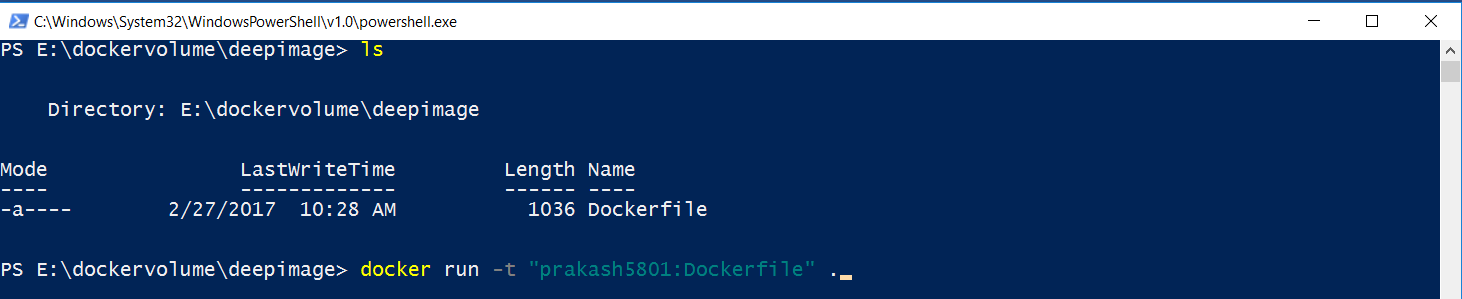Building the docker image

1. Access the image using the `docker images` command as follows: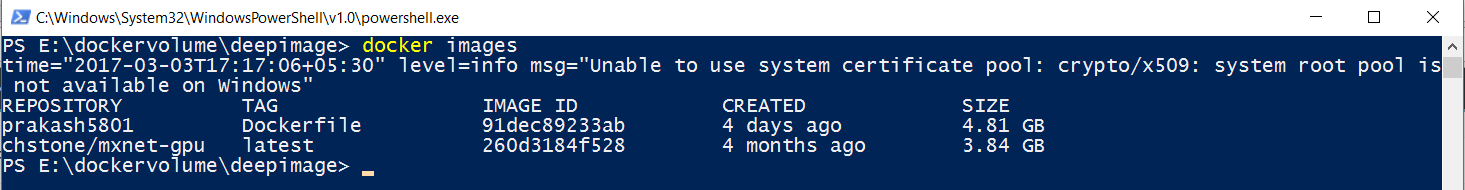View docker images

1. Docker images can be executed using the following command:
`docker run -it -p 8888:8888 -p 54321:54321 <<IMAGE ID>>`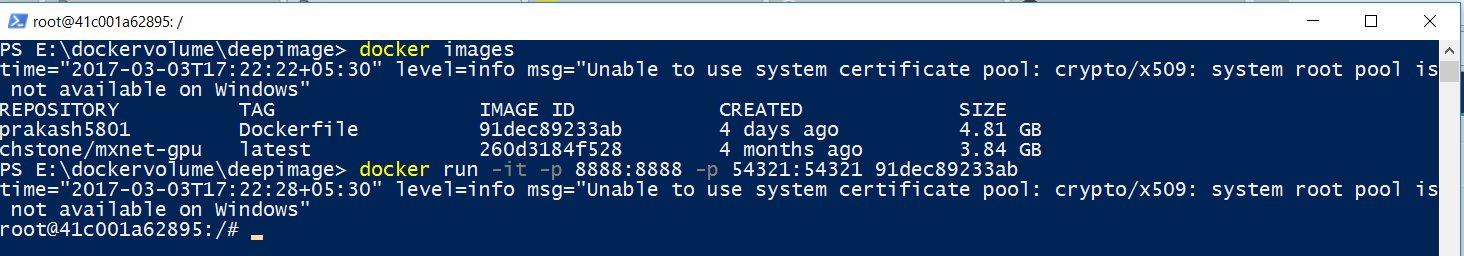Running a Docker image

Here, the option -i is for interactive mode and -t is to allocate --tty. The option -p is used to forward the port. As we will be running Jupyter on port `8888` and H2O on `54321`, we have forwarded both ports to accessible from the local browser.

### There's more...

More options for Docker can be checked out using `docker run --help`.

• Dr. PKS Prakash

Dr. PKS Prakash is a data scientist and author.

He has spent the last 12 years in developing many data science solutions in several practical areas in healthcare, manufacturing, pharmaceuticals, and e-commerce. He currently works as the data science manager at ZS Associates. He is the co-founder of Warwick Analytics, a spin-off from University of Warwick, UK. Prakash has published articles widely in research areas of operational research and management, soft computing tools, and advanced algorithms in leading journals such as IEEE-Trans, EJOR, and IJPR, among others. He has edited an article on Intelligent Approaches to Complex Systems and contributed to books such as Evolutionary Computing in Advanced Manufacturing published by WILEY and Algorithms and Data Structures using R and R Deep Learning Cookbook, published by PACKT.

Browse publications by this author
• Achyutuni Sri Krishna Rao

Achyutuni Sri Krishna Rao is a Data Scientist, a Civil Engineer and an Author. He has spent last 4 years in developing many data science solutions to solve problems from leading companies in healthcare, pharmaceutical and manufacturing domain. He is working as Data Science Consultant at ZS Associates.

Sri Krishna’s background involves a masters in Enterprise Business Analytics and Machine Learning from National University of Singapore, Singapore. His other educational background involves a Bachelor’s from National Institute of Technology Warangal, India.

Sri Krishna has published widely in research areas of civil engineering. He has contributed in a book titled “Algorithms and Data Structures using R” published by PACKT.

Browse publications by this author
Latest Reviews (2 reviews total)
The book is interesting to read
The epub-version crashes at least when trying to use with Google Play reader